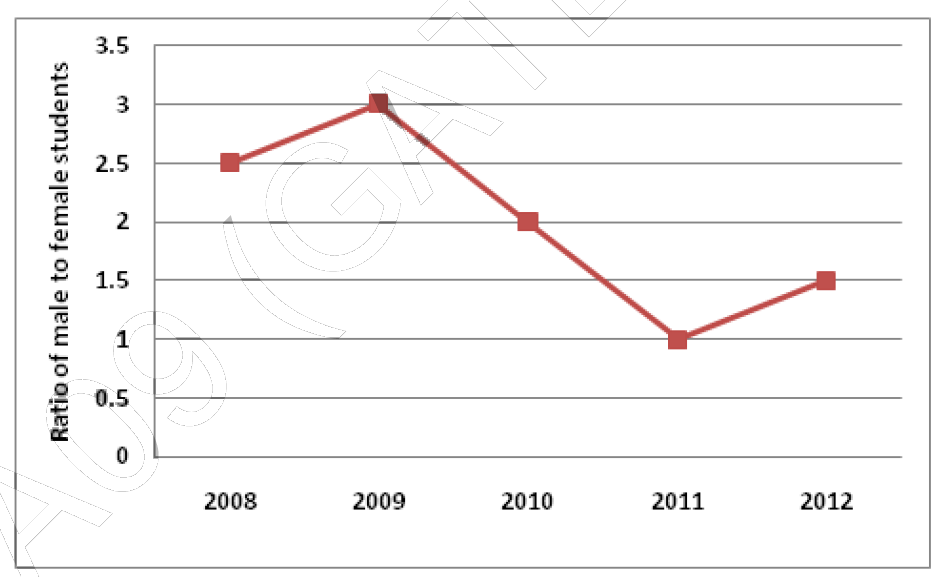Related Articles

# GATE | GATE-CS-2014-(Set-3) | Question 65

• Last Updated : 28 Jun, 2021

The ratio of male to female students in a college for five years is plotted in the following line graph. If the number of female students in 2011 and 2012 is equal, what is the ratio of male students in 2012 to male students in 2011?(A) 1:1
(B) 2:1
(C) 1.5:1
(D) 2.5:1

Explanation:

```Number of female students in 2011 and 2012 are equal.
Suppose they are x.

Now,
Males students in 2011 = Female students in 2011
= x (as the ratio is 1)

And in 2012, Male students / Female students =
1.5(Given)

Hence Male students in 2012 = 1.5 * Female students
= 1.5 * x

Now ratio of Male students in 2012 to Male students
in 2011 = ( 1.5 * x ) / x
= 1.5 / 1
i.e. 1.5 : 1

Hence C is the answer. ```

Attention reader! Don’t stop learning now.  Practice GATE exam well before the actual exam with the subject-wise and overall quizzes available in GATE Test Series Course.

Learn all GATE CS concepts with Free Live Classes on our youtube channel.

My Personal Notes arrow_drop_up